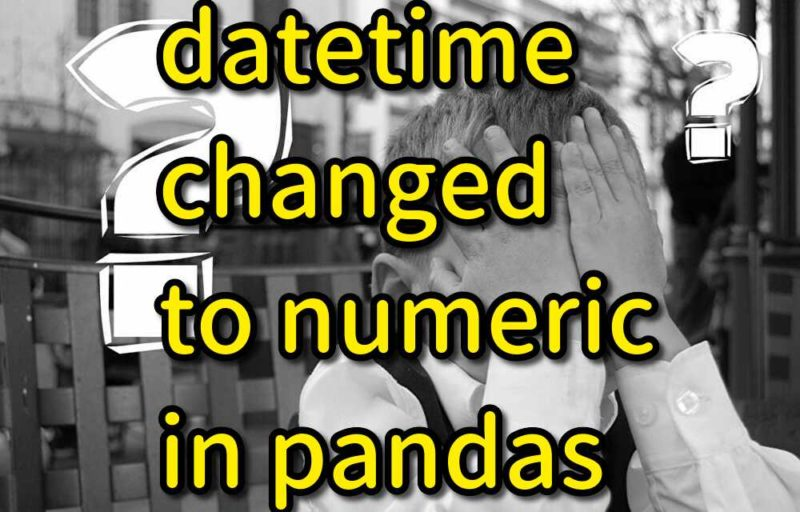# Why datetime value is converted to numeric in pandas?Strange thing was happened.
I set datetime value in Pandas Dataframe.
But it was changed to numeric value in next moment.
It was not a magic, so I would like show you why the datetime value was converted to numeric.

## datetime value is converted to numeric

I describe why datetime value is converted to numeric with using following codes.

### Initialize

First, I define Dataframe.

```import pandas as pd
import numpy as np
import datetime

cols = ["c1", "c2"]
vals = np.array([[1,2], [4,5]])
df = pd.DataFrame(vals, columns=cols)
print(df)

#    c1  c2
# 0   1   2
# 1   4   5
```

Then add a new column for datetime value.

```# Add new column
df["hoge"] = None
print(df)
#    c1  c2  hoge
# 0   1   2  None
# 1   4   5  None
```

### (Failure) Update by sliced Dataframe

Next, I sliced Dataframe and set datetime value (2019/11/28).
After that I updated original Dataframe by sliced Dataframe.
Then datetime value was converted numeric value `1574899200000000000` .

```# Update by sliced data
df_slice = df[df["c1"] > 3]
df_slice["hoge"] = datetime.datetime(2019,11,28)
df["hoge"].update(df_slice["hoge"])
print(df)

#    c1  c2                 hoge
# 0   1   2                 None
# 1   4   5  1574899200000000000
```

### (Solution) Convert column to datetime format

When I thought about cause of conversion, I thought that it may be caused by column format.
Original column format is not datetime. So it was converted in update.
In order to confirm the hypothesis, I converted the added column to datetime. After that I updated and I could see date value. But it seemed just a date value, not a datetime value.

```# Convert column before update
df["hoge"] = pd.to_datetime(df["hoge"])
df_slice = df[df["c1"] > 3]
df_slice["hoge"] = datetime.datetime(2019,11,28)
df["hoge"].update(df_slice["hoge"])
print(df)

#    c1  c2       hoge
# 0   1   2        NaT
# 1   4   5 2019-11-28
```

### (Solution2) Use index and loc instead of updatePython says "Do not set value directly. Use `loc`."
So I use index and loc instead of `update`.
Then datetime value was set in original Dataframe.

A value is trying to be set on a copy of a slice from a DataFrame.
Try using .loc[row_indexer,col_indexer] = value instead

```# Use index and loc instead of update
df_slice_index = df[df["c1"] > 3].index
df.loc[df_slice_index,"hoge"] = datetime.datetime(2019,11,28)
print(df)

#    c1  c2                 hoge
# 0   1   2                 None
# 1   4   5  2019-11-28 00:00:00
```

## Finally

Why datetime value is converted to numeric in pandas?
It is because original column format is not datetime.
So it is converted to numeric value when it is updated.

Solutions are following.

• Convert column to datetime
• Use `loc` instead of `update`

コチラもオススメKRSW

Translate »